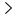ferris blogs stuff

Technical ramblings and other stuff

ferris blogs stuffStochastic rounding

23 Mar 2019

Stochastic rounding

So here’s a little quickie based on something I encountered in my new job :) .

The main motivation is that you’d like to work with lower precision numbers than you typically work with for some reason (typically to reduce storage for some model and ideally do faster calculations). But ofc to do that you need to drop some precision, which involves rounding.

Often times this rounding ends up being a floor or towards-zero round, sometimes a nearest round, but whichever you choose you’re still losing fractional information.

So the idea behind a stochastic round is that you use some random number that decides whether you round up or down, instead of always doing one or the other or taking the closest integer. The critical detail here is that the distribution of that number matches that of the fractional part of the number you’re rounding.

eg. if you round 1.3 to the nearest integer 100 times, you round up 30% of the time, down 70%.

likewise if you round 1.6 100 times, you round up 60% of the time, down 40%.

So if you just sum up that rounded value 100 times, you get the same result as if you had done the adds with higher precision and then rounded the normal way, which is a really cool idea actually! Of course you still get some cumulative error over time in practical use cases, but it actually happens to work out pretty well and tends to be noticeably better than just dropping the extra precision.

So naturally, I had to try this in the probability update in my c64 packer, where it should apply nicely since it only uses 8 bits for probabilities and updates. :)

In that packer I currently update probabilities with something like this:

let adjustment = error/16 (up or down depending on bit)

This is very simplified (I already wrote about it in much more detail here) but the point is that I have 4 bits of fractional precision and 4 bits of whole precision for the update (using 8 bit probs), and currently I do the /16 as 4 right shifts, which is essentially a floor divide (and a towards-zero divide since the adjustment is never negative).

To get stochastic rounding, I need some kind of super cheap PRNG that respects the distribution of the fractional bits. After some thinking I came up with something very simple, yet pretty effective. The idea is to use the fractional bits of the number I’m rounding as a decision threshold. If we generate a pseudo-random value with the same number of bits, we can just compare the value with the fractional bits. If the value is less than the fractional bits, we round up; otherwise, we round down. As long as the PRNG we choose has pretty even distribution over the possible fractional bit values, we should achieve decent stochastic rounding.

For the PRNG, I chose to use a rotating value in [0, 16) that I add 9 to each time (something close to golden ratio for 16) so that this cycles within this interval in a semi-random fashion where each value has equal probability. I got this idea from how FSE state tables are generated, and it’s ofc not a great PRNG, but it’s very cheap (especially on 6502) both in terms of performance and code complexity, so it made for a good test.

This kind of probability update with stochastic rounding looks something like this:

stochastic_counter = (stochastic_counter + 9) & 0x0f
let adjustment = error/16
if stochastic_counter < fract(adjustment) {
} else {
}